Performance Evaluation of PVT Mixed Mode Dryer with Load Condition | OMICS International
Journal of Fundamentals of Renewable Energy and Applications

# Performance Evaluation of PVT Mixed Mode Dryer with Load Condition

Shyam* and GN Tiwari

Centre for Energy Studies, Indian Institute of Technology Delhi, Hauz Khas, New Delhi, India

Corresponding Author:
Shyam
Centre for Energy Studies
Indian Institute of Technology Delhi
Hauz Khas, New Delhi, India
Tel: +91 7838959195
Fax : +91 11 26591251
E-mail: [email protected]

Received Date: November 15, 2013; Accepted Date: October 14, 2014; Published Date: October 22, 2014

Citation: Shyam, Tiwari GN (2014) Performance Evaluation of PVT Mixed Mode Dryer with Load Condition. J Fundam Renewable Energy Appl 4:141. doi:10.4172/2090-4541.1000141

Copyright: © 2014 Shyam, et al. This is an open-access article distributed under the terms of the Creative Commons Attribution License, which permits unrestricted use, distribution, and reproduction in any medium, provided the original author and source are credited.

Visit for more related articles at Journal of Fundamentals of Renewable Energy and Applications

#### Abstract

In this communication, an attempt has been made to evaluate the performance of mixed mode dryer consists of PVT air collectors and wooden made drying chamber with load condition. Based on energy balances of PVT air collectors and conventional collector, an expression for outlet air temperature of PVT air collector has been derived. Further by using the rate of thermal energy available from PVT air collector, an analytical expression for crop temperatures has also been derived in terms of design and climatic parameters of New Delhi and experimentally validated. The validation of analytical model has been carried out for cauliflower with thin layer drying for last day of drying with reasonable assumptions. It has been observed that the drying time for a half kilogram of cauliflower is achieved in 21 hours due to larger size of the samples. The annual overall thermal energy and exergy were 396.48 kWh and 46.52 kWh respectively. The energy payback time (EPBT) was increased from 3.17 years on thermal energy basis to 27.03 years on exergy basis for mixed mode dryer. The energy production factor and life cycle conversion efficiency were found to be smaller on exergy basis compared to thermal energy basis. For a life time of 30 year the carbon credits earned on overall thermal energy basis was \$ 727.9 and on overall exergy basis it was \$ 85.5.

#### Keywords

Mixed mode dryer; PVT air collector; Energy matrices

#### Introduction

The photovoltaic thermal (PVT) systems utilize both the thermal energy available from the sun as well as the electrical energy produced by the photovoltaic (PV) module. Extraction of thermal energy from the PVT system by fluid (water/air) improves the electrical efficiency which in turn enhances the energy and exergy efficiency of the PVT systems. The hot water/air can be utilized for various applications for example, to heat up living space, greenhouse, solar dryers, solar stills, etc . The use of PV power renders the entire system fully independent of grid electricity making it more reliable. Figure 1 shows the classification of solar dryers. Different types of solar dryers basically differ in the methods used for transferring heat to the product. In general, solar dryers can be classified into two broad categories: passive (natural convection) solar dryers and active (forced convection) solar dryers [2-6].

One of the most viable solutions for the population-food imbalance is to reduce the food losses drastically, which occur throughout the food production, harvest, postharvest and marketing [7-9].

Drying of agricultural product is one of the important postharvest operations to save the grain from postharvest losses. Among the many factors which affect the rate of drying, the air temperature and air velocity are the most important and energy consuming factors which make the drying process as an energy-intensive operation. The use of energy for drying purposes in the developed countries amounts to be around 8-12 percent of the total demand of primary energy. The high energy consumption causes substantial ecological burden of the agricultural production and direct effect on drying operation and thus, the direct effect on market price of the dried product . The solar drying is quite feasible in tropical country like India where average solar radiation varies between 4-7 kWh/m2 for different part of country. For the rural area where the grid power is unavailable the PVT systems are most suitable option for controlled drying purpose.

The working principle of PVT collectors is similar to flat plate solar collectors. Since the PVT collectors are integrated with the PV module, it generates electricity from incident solar radiation . In recent years there are rapid increments in prices of fossil fuels in the international market which make energy more costly but in this duration the cost of PV modules reduced significantly due to the technological advancements in PV technologies. It is advantageous to use PVT systems . Interest in zero energy building and green buildings and the possibility of integrating PVT systems into buildings and environmental concerns prompt us to use the PVT system. Dubey et al. [13,14] developed an expression for the electrical efficiency of the PVT module of PVT air collectors as a function of climatic and design parameters, and applied it to several cases (glass-to-glass PV module with and without ducts, glass-to-tedlar PV module with and without ducts). The highest electrical efficiency and outlet air temperature were shown to be achieved for the case of a glass-to-glass PV module with a duct. Annual thermal outputs as well as electrical and energy efficiencies were evaluated for five cities in India. Tiwari et al. [15-17] have conducted extensive studies on PVT air and water collectors, involving numerical simulation, experimentation, prototype design and testing procedure development. It was demonstrated that the glazed PVT air collector without tedlar performs better than other designs, and that the system with a small number of in-series collectors yields a higher output than an equivalent single collector.

Energy matrices are a tool of any electrical based system to optimize the unit from economical point of view. The energy matrices have been determined on overall thermal energy basis and overall exergy basis. On the basis of exergy analysis of PV module, Slesser and Houman  have reported that the energy payback time (EPBT) of PV module to be 40 years. A similar study has been carried out by Hunt  and it was reported that energy payback time is 12 years of PV module which are in accordance with the results by Kato et al.  for crystalline silicon (c-Si) solar cell module. Alsema and Nieuwar  have attempted to forecast energy payback time for mono-crystalline solar for year 2020, by considering the improved technology in future with higher electrical efficiency of the solar cell; energy payback time ,which is 5-6 years, while Prakash and Bansal , got energy payback time, 1.5-2 years.

Further it has been found that simultaneous generation of thermal and electrical energy (PVT) from PV system is the best option to reduce the EPBT of PV system. Raman and Tiwari  have carried out life cycle analysis in terms of cost/kWh of a photovoltaic thermal (PVT) air collector for different Indian climatic conditions. The study reveals that hot and dry climate are most economical.

Mortaza et al.  extensively reviewed the energy analysis of drying processes and systems and concluded that exergetic analysis provides a tool for a more realistic view between energy losses to the environment and internal irreversibility in the process. The optimization of drying processes on energy basis quantifies the sources of inefficiency which in turn gives a clear understanding of the process for optimization of drying conditions and reduction of ill impacts of drying system on environment. Currently, the concept of exergoeconomics is increasing amongst the researchers which combine the energy and economic concepts. The exergoeconomic concept is a tool to evaluate the costs of inefficiencies or the costs of individual process streams, including intermediate and final products.

#### Experimental Set Up and Working Principle of Mixed Mode Dryer

The main components of a solar drying system are solar air heater and drying unit. Solar air heater consists of PV panel and flat plate collector. It consists of PV panel (Glass-to-glass) for converting solar radiation into electricity. This panel has been integrated with flat plate collector at an inclination of 30° for receiving maximum solar radiation. Solar air heater is used for heating the ambient air available at the inlet point of air duct. The schematic diagram of mixed mode dryer is shown in Figure 2. In this case the drying unit is connected to the air heater. The incoming solar radiations fall on the PV module, which converts solar radiation into electricity and the collector absorbs the radiation, convert it into thermal energy. This energy is utilized to heat air, which is supplied to the drying unit for reduction of moisture content of agricultural commodity. The hot air from the air heater is extracted by a 12V DC fan provided at the outlet of air heater. It is operated by the electricity generated from the PV module. It supplies air into the drying chamber where materials to be dried, placed into the three trays.

#### Thermal Modelling

To develop thermal modeling following assumptions have been made:

(i) Perfectly insulated drying chamber i.e. no loss from the side walls of the drying chamber.

(ii) Heat capacities of the metals are negligible.

(iii) Thin layer drying approximation.

(iv) As the mass of the crop in each tray is very small, porosity is very large and therefore it is assumed that the temperature of crop in each tray is approximately equal.

(v) Modeling has been carried out for dried product placed in single tray.

Energy balance for the semitransparent PV module and duct

The energy balance for the system has been written for each unit of the system by considering the small elemental area bdx in the direction of flow of the air through air duct as shown in Figure 3.

For Module: Rate of Solar energy available on cell=The rate of overall heat lost from top surface of the cell to ambient+ The rate of overall heat transferred from back surface of the cell to the flowing fluid + The rate of electrical energy produced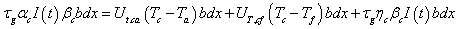(1)

For the duct: Rate of Solar energy available on the Plate=The rate of overall heat transferred from top surface of the plate to the flowing fluid + The rate of overall heat lost from back surface of the plate to ambient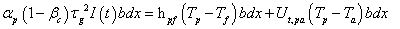(2)

The heat gain by the fluid is sum of the heat gained from the bottom surface of the PV module and the heat gained from top surface of the plate of the duct. The heat gain by the fluid is given as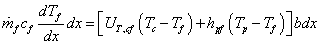(3)

From equation (1), (2) and (3) one can get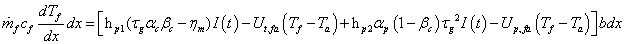on solving above equation with boundary conditions at x=0, Tf =Tfi and At x=Lm, Tf =Tf01, one can find expression for Tf01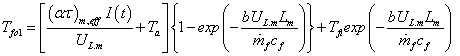(4)

Energy balance for the conventional collector: Energy balance for conventional collector is given as following Rate of solar energy available on the blackened plate=The rate of overall heat transferred from top surface of the plate to the flowing fluid + The rate of overall heat lost from back surface of the plate to ambient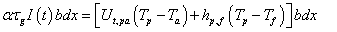(5)

The rate of thermal energy carried out by the flowing fluid is given by,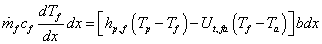(6)

From equation (5) one can get the expression of Tp and on putting the value of hp,f (Tp–Tf) into (6) then by solving the differential equation by applying boundary conditions, at x=0, Tf=Tfo1 and At x=Lc, Tf =Tf02 expression for Tf02 can be obtained and given as following,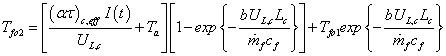(7)

on putting the value of Tf01 we have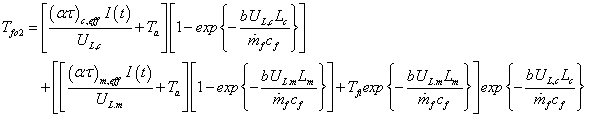(8)

Energy balance for drying chamber: The rate of available thermal energy from the side walls of drying chamber plus the rate of available thermal energy from the flowing fluid is utilized to raise the temperature of crop and the remaining heat will be lost to the drying chamber.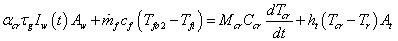(9)

In steady state condition, the heat lost from the tray will be equal to the heat carried away by the flowing fluid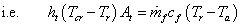(10)

using equations (8), (9) and (10) and putting on one can get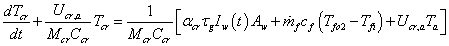(11)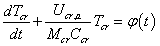(12)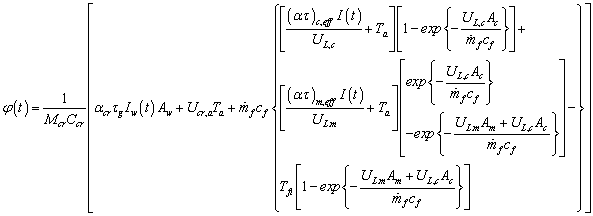(13)

solution of equation (12) is given as,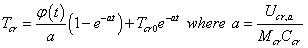(14)

The values of different designing parameters are listed in Table 1, which were used in the numerical calculation of outlet temperature, thermal energy and electrical energy.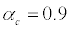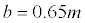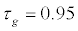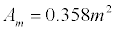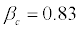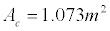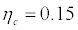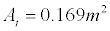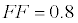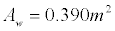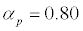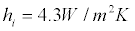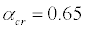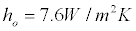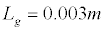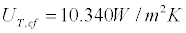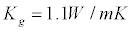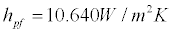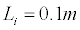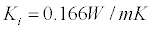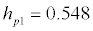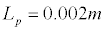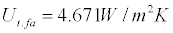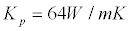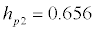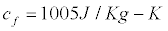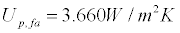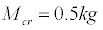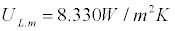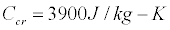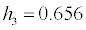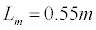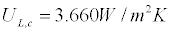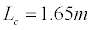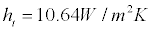Table 1: Values of design parameters used in numerical computation of crop temperature.

#### Methodology

The annual useful energy was calculated on overall thermal energy and overall energy basis. The rate of thermal energy available from flat plate collector was calculated by the following equations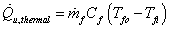(15)

The Tfo is the outlet air temperature of PVT mixed mode dryer.

The net hourly electrical energy of PVT air collectors of mixed mode dryer in terms of load current and load voltage has been calculated as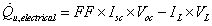(16)

FF is the fill factor or power factor of PVT air collector of mixed mode dryer.

The overall thermal energy was calculated by the following equation.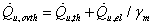(17)

where m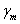=0.38 for good quality of Indian coal and it has lower value for other quality of coal. The range of mis varying between 0.20-0.40. The electrical energy has been converted to equivalent thermal by using electric power generation efficiency as 0.38 for a conventional power plant .

The overall exergy was calculated using following equation.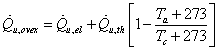(18)

The exergy analysis includes accounting the total exergy inflow, exergy outflow and exergy destructed from the system.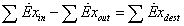(19)

or,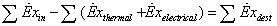Where exergy of incident solar radiation is given by Petela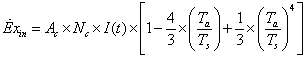(20)

where Ac is area of collector and Ts is the Sun temperature in Kelvin.

For average (i) annual solar energy I =400 W/m2, (ii) sunshine hour=6 hour, (iii) Nc=1 and (iv) Ac=1.43 m2, annual solar thermal radiation available on PVT mixed mode dryer is given by

Eannual, sol=400×1.43× 6×268×10-3=919.77 kWh (21)

Now, annual solar exergy available on PVT mixed mode dryer is given by

Exannual, ex= 919.77× 0.934=859.07 kWh (22)

The following methodology has been used to calculate the overall thermal energy and overall exergy, and carbon credit earned by PVT mixed mode dryer.

Overall thermal energy

The annual overall thermal energy has been calculated in the following steps

(i) The hourly variation of thermal energy was calculated for each day of first cycle (November 19-21, 2012) of the month using equation (15).

(ii) The hourly data’s of each day have been then added to get daily thermal energy in kWh.

(iii) Further the daily thermal energy was added to get total thermal energy for each cycle means for three days.

(iv) The total thermal energy for each cycle was multiplied by number of cycle in the month to get monthly thermal energy.

(v) The above calculations have been repeated for each month.

(vi) Finally, monthly thermal energy in kWh was added to get annual thermal energy in kWh.

To get the annual overall thermal energy equation (17) was used for this, the annual electrical energy was converted to equivalent annual thermal energy using equation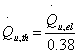and then the equivalent annual thermal energy was added to the above calculated annual thermal energy.

Overall exergy

The hourly variation of electrical energy was calculated for each day of first cycle (November 19-21, 2012) of the month using equation (16), and then the same procedure has been adopted to calculate the annual electrical energy. Further to get the annual overall exergy equation (18) was used for this, the annual thermal energy was converted to equivalent annual electrical energy by using equation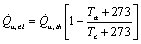.

Thereafter the equivalent electrical energy was added to the previously calculate electrical energy.

Energy matrices

It was assumed in the present work that there was uniform thermal/ electrical energy for all clear sky condition. The energy matrices PVT mixed mode dryer were determined by considering the total energy required for individual components with their manufacturing energy needs. The breakup of embodied energy of each components of fabrication of PVT mixed mode dryer has been tabulated in Table 2.

S. N. Items Weight
(kg)
Energy density
(kWh/kg)
Total embodied energy (kWh)
1. Glass 14.00 7.28 101.92
2. Steel 10.00 8.89 88.9
3. Paint 1.00 25.11 25.11
4. Rubber gasket and polyethylene sheet 1.00 25.64 25.64
5. Fittings (Nuts bolt with washer, steel screws and rivets etc.) 1.00 8.89 8.89
6. Aluminum sheet 10.00 55.28 552.8
7. Wood material 20.00 2.89 57.8
8. PV module (glass to glass; size: (0.6×0.55×0.01m) 1 No. 369.5 369.5
9. DC fan 1 No. 26.83 26.83
Total     1257.39

Table 2: Calculation of embodied energy for PVT mixed mode dryer.

Energy payback time (EPBT): The EPBT is the total time required to recover the total energy which was spent to prepare the materials used for fabrication of a system. EPBT has been calculated by taking the ratio of the total energy consumed in production and installation of the system (Eembodied) to the total energy output (Eout).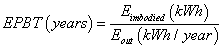(23)

Energy production factor (EPF): The overall energy performance of the PVT system can be evaluated by comparing the total energy input with the total energy output. The energy production factor has been calculated by the following formula.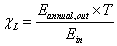(24)

Life cycle conversion efficiency (LCCE): The life cycle conversion efficiency is the net energy productivity of the system with respect to the solar input (radiation) over the life time of the system, (T, years). The LCCE has been calculated by following formula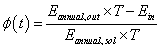(25)

Carbon credit earned by PVT mixed mode dryer

Total carbon credits earned by PVT mixed mode dryer have been calculated on the life time basis considering both aspects of energy namely overall thermal energy and overall exergy. To calculate the carbon credit earned, following procedure has been adopted:

Overall thermal energy basis: (i) The cost of energy produced per annum has been calculated by multiplying overall thermal energy per annum with unit cost of electricity @ \$ 0.1 per unit of electricity.

(ii) If we consider the inefficiencies in the domestic appliances to be 20 % and the transmission and distribution losses equal to 40 % for Indian condition then for the unit power to be used by the consumer the power generated in the plant should be 2.08 units. Again the average carbon dioxide (CO2) equivalent intensity of electricity generation from coal is approximately 0.982 kg of CO2/kWh at power plant [27, 28]. Thus, for unit power consumption by the consumer the amount of CO2 emission is 2.08×0.982=2.04 kg.

(iii) The reduced amount of CO2 emitted per annum has been calculated by multiplying annual overall thermal energy and amount of CO2 emitted (in tonnes) per unit power generated.

(iv) If the reduced amount of carbon is traded @ \$ 30/tCO2e, then cost of the reduced amount of carbon emission by PVT mixed mode dryer can be obtained by multiplying the reduced amount of carbon dioxide emission per annum in ton and \$ 30.

(v) By multiplying the above calculated cost in point (iv) with the life time of the PVT mixed mode dryer one can get the credits earned by the PVT mixed mode dryer on life time basis.

Overall exergy basis: Same methodology has been adopted to calculate to carbon credit earned by PVT mixed mode dryer on overall exergy basis as explained for overall thermal energy basis. Overall exergy will be taken in place of overall thermal energy gain in above said methodology.

#### Results and Discussion

The initial weight, final weight and amount of water evaporated on first day from each trays and open sun drying have shown in Figure 4. From Figure 4 one can see that the amount of water mass removed from tray1(bottom tray) is maximum due to direct contact of hot air from PVT air collectors and minimum from tray 3 (top tray). However, the moisture removed from open sun drying is lower than the moisture removed from tray 3 (top tray) due to higher air temperature even in top tray in comparison with open case. Further it is to be noted that moisture remained higher in open sun drying case as expected. On second day of drying the amount of mass evaporated from each tray is reduced in comparison with first day of drying due to less amount of water in crop available for removal. On third day of drying amount of water removed becomes same in all cases.

Hourly variation of solar radiation and ambient temperature with load condition has been shown in Figure 5 for a typical day November 19, 2012. From the figure one can observe that as the intensity of radiation increases with time the ambient air temperature increases and reaches maximum around 2 pm, then decreases.

Corresponding to the intensity variations of Figure 5, hourly variation of ambient air temperature, inlet air temperature, outlet air temperature and the solar cell temperature has been shown in Figure 6. Here the solar cell temperature has maximum value compared to outlet and inlet temperature because of the higher absorptivity of solar cell. The inlet air temperature is higher than ambient air temperature due to radiation effect from surrounding at inlet of mixed mode dryer.

Figure 7 shows the hourly variation of solar cell temperature and electrical efficiency of solar cell for load condition. It can be seen that as the solar cell temperature increases with time, hourly electrical efficiency decreases due to collision between electrons in depletion region which is expected.

Figure 8 gives the information about net power generated from mixed mode dryer with load condition, which can be used for other purposes than running the fan. One can observe that for a typical day average 20 W per hour can be stored or used for other application simultaneously with drying.

The experimental validation has been done on the basis of thermal model developed earlier with approximation mentioned in section 3. The experimental validation has been done for the last day of drying when the entire product was kept on the single (middle tray) tray. Equation (14) has been used for computation by using MATLAB 7 for given design parameters and climatic parameters. The experimental and theoretical values of outlet air temperature of mixed mode dryer have been shown in Figure 9. It has been observed that there is fair agreement between experimental and theoretical value with correlation coefficient r=0.997 and e=5.157.

The overall thermal energy was calculated by using equation (17). Monthly variation of overall thermal energy is shown in Figure 10. It is observed that the rate of overall thermal energy without load condition is more than with load condition due to the storage effect in load condition. The rate of overall exergy was calculated by using equation (18). Monthly variation of overall electrical energy is shown in Figure 11. It is observed that the rate of overall electrical energy with load condition is more than without load condition due to the more available solar radiation in a particular month.

To compare the useful overall thermal energy and useful overall exergy data’s without load condition are also shown in Figure 10 and Figure 11.

The energy payback times for PVT mixed mode dryer on overall thermal energy basis and on overall exergy basis are 3.17 years and 27.03 years respectively. The energy production factor and life cycle conversion efficiency for different life time of the PVT mixed mode dryer are shown in Tables 3 and Table 4 respectively. From the Tables 3 and 4 it is clear that for the higher life time the energy production factor and the life cycle conversion efficiency are higher on overall thermal energy basis and overall exergy basis. But the values of energy production factor and the life cycle conversion efficiency in case of overall thermal energy basis are higher than that of the overall exergy basis.

S. No. Basis Life time of PVT mixed mode dryer
5 yrs 10 yrs 15 yrs 20 yrs 25 yrs 30 yrs
1 Overall thermal Energy basis 1.58 3.15 4.73 6.31 7.89 9.46
2 Overall exergy basis 0.19 0.37 0.55 0.74 0.92 1.11

Table 3: Energy production factor of PVT mixed mode dryer for different lifetime.

S. No. Basis Life time of  PVT mixed mode dryer
5 yrs 10 yrs 15 yrs 20 yrs 25 yrs 30 yrs
1 Overall thermal Energy basis 0.253 0.389 0.435 0.458 0.472 0.481
2 Overall exergy basis -0.238 -0.092 -0.044 -0.019 -0.005 0.005

Table 4: Life cycle conversion efficiency of PVT mixed mode dryer for different lifetime.

For different life time, the carbon credits earned by the PVT mixed mode dryer are listed in Table 5. One can observed from the Table 5 that the carbon credits earned on overall thermal energy basis is higher than that earned on overall exergy basis. More carbon credits are earned for higher life time of the PVT mixed mode dryer.

S. No. Basis Life time of  PVT mixed mode dryer
5 yrs 10 yrs 15 yrs 20 yrs 25 yrs 30 yrs
1 Overall thermal Energy basis 121.3 242.6 364.0 485.3 606.6 727.9
2 Overall exergy basis 14.2 28.5 42.7 56.9 71.2 85.4

Table 5: Carbon Credits earned (in \$) by the PVT mixed mode dryer for different lifetime.

#### Conclusion

The mixed mode dryer shows behavior similar to the flat plate collector because it is designed on the thermal energy point of view. It is observed that there is better agreement between theoretical and experimental observations with load condition for crop temperature of mixed mode dryer. The energy payback time (EPBT) is increased from 3.17 years on thermal energy basis to 27.03 years on exergy basis for mixed mode dryer. The energy production factor and life cycle conversion efficiency are smaller on exergy basis compared to thermal energy basis. For life span of 30 years of PVT mixed mode dryer the carbon credit earned on overall thermal energy basis and on overall exergy basis are \$ 727.9 and \$ 85.4 respectively.

#### References

Select your language of interest to view the total content in your interested language

### Article Usage

• Total views: 13439
• [From(publication date):
November-2014 - Dec 12, 2019]
• Breakdown by view type
• HTML page views : 9448Can't read the image? click here to refresh